Courses

# The Force Method of Analysis: Beams - 1 Civil Engineering (CE) Notes | EduRev

## Civil Engineering (CE) : The Force Method of Analysis: Beams - 1 Civil Engineering (CE) Notes | EduRev

The document The Force Method of Analysis: Beams - 1 Civil Engineering (CE) Notes | EduRev is a part of the Civil Engineering (CE) Course Structural Analysis.
All you need of Civil Engineering (CE) at this link: Civil Engineering (CE)

Instructional Objectives

After reading this chapter the student will be able to

1. Solve statically indeterminate beams of degree more than one.
2. To solve the problem in matrix notation.
3. To compute reactions at all the supports.
4. To compute internal resisting bending moment at any section of the continuous beam.

Introduction

In the last lesson, a general introduction to the force method of analysis is given. Only, beams, which are statically indeterminate to first degree, were considered. If the structure is statically indeterminate to a degree more than one, then the approach presented in the previous example needs to be organized properly. In the present lesson, a general procedure for analyzing statically indeterminate beams is discussed.

Formalization of Procedure

Towards this end, consider a two-span continuous beam as shown in Fig. 8.1a. The flexural rigidity of this continuous beam is assumed to be constant and is taken as EI. Since, the beam is statically indeterminate to second degree, it is required to identify two redundant reaction components, which need be released to make the beam statically determinate.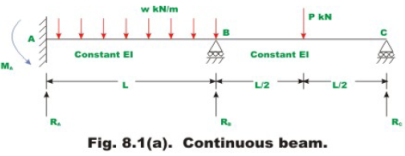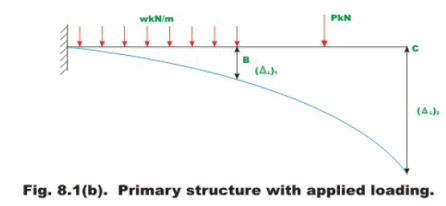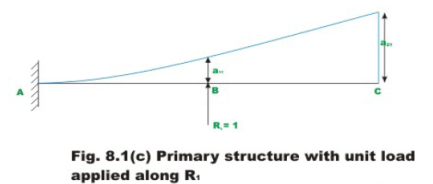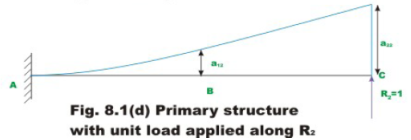The redundant reactions at A and B are denoted by R1 and R2 respectively. The released structure (statically determinate structure) with applied loading is shown in Fig. 8.1b. The deflection of primary structure at B and C due to applied loading is denoted by (ΔL)1 and (ΔL)2 respectively. Throughout this module (ΔL)i notation is used to denote deflection at ith redundant due to applied loads on the determinate structure.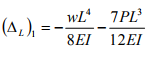(8.1a)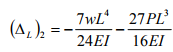(8.1b)

In fact, the subscript 1 and represent, locations of redundant reactions released. In the present case RA (=R1) and RB (=R2) respectively. In the present and subsequent lessons of this module, the deflections and the reactions are taken to be positive in the upward direction. However, it should be kept in mind that the positive sense of the redundant can be chosen arbitrarily. The deflection of the point of application of the redundant should likewise be considered positive when acting in the same sense.
For writing compatibility equations at B and C, it is required to know deflection of the released structure at B and C, due to external loading and due to redundants. The deflection at B and C due to external loading can be computed easily. Since redundants R1 and R2 are are not known, in the first step apply a unit load in the direction of R1 and compute deflection, a11 at B, and deflection, a21 at C, as shown in Fig.8.1c. Now deflections at B and C of the given released structure due to redundant R1 are,

R)11 = a11 R1               (8.2a)

R)21 = a21 R1                    (8.2b)

In the second step, apply unit load in the direction of redundant R2 and compute deflection at B (point 1), a12 and deflection at C, a22 as shown in Fig 8.1d. It may be recalled that the flexibility coefficient aij is the deflection at i due to unit value of force applied at j. Now deflections of the primary structure (released structure) at B and C due to redundant R2 is

R)12 = a12 R2                     (8.3a)

R)22 = a22 R2               (8.3b)

It is observed that, in the actual structure, the deflections at joints B and C is zero. Now the total deflections at B and C of the primary structure due to applied external loading and redundants R1 and R2 is,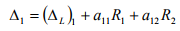(8.4a)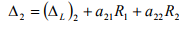(8.4b)

The equation (8.4a) represents the total displacement at B and is obtained by superposition of three terms:

1) Deflection at B due to actual load acting on the statically determinate structure,
2) Displacement at B due to the redundant reaction R1 acting in the positive direction at B (point 1) and
3) Displacement at B due to the redundant reaction R2 acting in the positive direction at C.

The second equation (8.4b) similarly represents the total deflection at C. From the physics of the problem, the compatibility condition can be written as,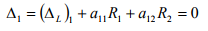(8.5a)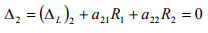(8.5b)

The equation (8.5a) and (8.5b) may be written in matrix notation as follows,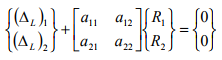(8.6a)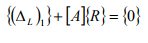(8.6b)

In which,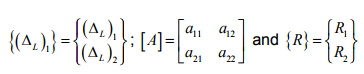Solving the above set of algebraic equations, one could obtain the values of redundants, R1 and R2.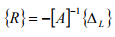(8.7)

In the above equation the vectors {ΔL} contains the displacement values of the primary structure at point 1 and 2, [A] is the flexibility matrix and {R} is column vector of redundants required to be evaluated. In equation (8.7) the inverse of the flexibility matrix is denoted by [A]-1. In the above example, the structure is indeterminate to second degree and the size of flexibility matrix is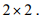In general, if the structure is redundant to a degree n, then the flexibility matrix is of the order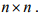To demonstrate the procedure to evaluate deflection, consider the problem given in Fig. 8.1a, with loading as given below

w = w ;                 P = wl                    (8.8a)

Now, the deflection (ΔL)1 and (ΔL)2 of the released structure can be evaluated from the equations (8.1a) and (8.1b) respectively. Then,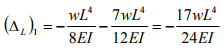(8.8b)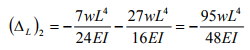(8.8c)

The negative sign indicates that both deflections are downwards. Hence the vector {ΔL} is  given by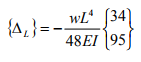(8.8d)

The flexibility matrix is determined from referring to figures 8.1c and 8.1d. Thus, when the unit load corresponding to R1 is acting at B, the  deflections are,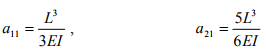(8.8e)

Similarly when the unit load is acting at C ,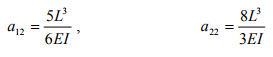(8.8f)

The flexibility matrix can be written as,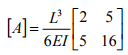(8.8g)

The inverse of the flexibility matrix can be evaluated by any of the standard method. Thus,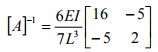(8.8h)

Now using equation (8.7) the redundants are evaluated. Thus,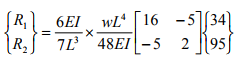Hence,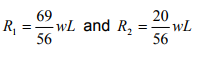(8.8i)

Once the redundants are evaluated, the other reaction components can be evaluated by static equations of equilibrium.

Offer running on EduRev: Apply code STAYHOME200 to get INR 200 off on our premium plan EduRev Infinity!

## Structural Analysis

30 videos|122 docs|28 tests

,

,

,

,

,

,

,

,

,

,

,

,

,

,

,

,

,

,

,

,

,

;